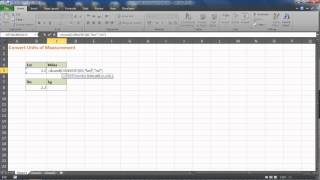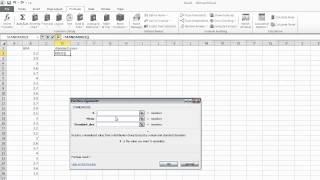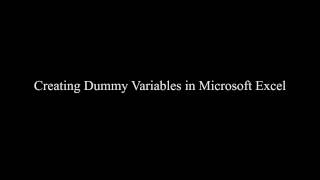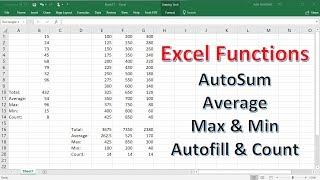Excel Tips & Tutorials:
Creating and Transforming Variables
 Suggest a Link Alphabetize Page Printer-Friendly List
 More Excel Tips & Tutorials: Select Page ... Getting Started with Excel Data Analysis with Excel Descriptive Statistics Creating and Transforming Variables Making Comparisons Graphing Relationships and Describing Patterns Random Assignment and Sampling Making Controlled Comparisons Foundations of Statistical Inference Hypothesis Tests with One and Two Samples Chi-Square and Analysis of Variance Correlation and Bivariate Regression Multiple Regression Analyzing Regression Residuals Logistic Regression Doing Your Own Political Analysis Advanced Political Analysis with Excel
Watch and Learn
Watch and Learn
Watch and Learn
Watch and Learn
 How to Convert Measurement UnitsUsing the EXCEL function (5:54)How to Use STANDARDIZE FunctionDemonstration with student GPAs (1:39)How to Create Dummy VariablesTaylor Smith demonstrates by converting categorical variable into dummy variables (7:25)How to Use Autosum, Average, Max, Min, Count & Autofill FunctionsFrom Organic Chemistry Tutor (6:46)How to Convert Measurement UnitsUsing the EXCEL function (5:54)How to Use STANDARDIZE FunctionDemonstration with student GPAs (1:39)